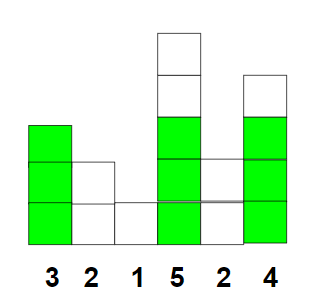Related Articles

# Largest square which can be formed using given rectangular blocks

• Last Updated : 24 Mar, 2021

Given an array arr[] of positive integers where each element of the array represents the length of the rectangular blocks. The task is to find the largest length of the square which can be formed using the rectangular blocks.

Examples:

Input: arr[] = {3, 2, 1, 5, 2, 4}
Output:
Explanation:
Using rectangular block of length 3, 5 and 4, square of side length 3 can be constructed as shown below:Input: arr[] = {1, 2, 3}
Output:

Approach:

1. Sort the given array in decreasing order.
2. Initialise maximum sidelength(say maxLength) as 0.
3. Traverse the array arr[] and if arr[i] > maxLength then increment the maxLength and check this condition for next iteration.
4. If the above condition doesn’t satisfy then break the loop and print the maxLength.

Below is the implementation of the above approach:

## C++

 `// C++ program for the above approach``#include ``using` `namespace` `std;` `// Function to find maximum side``// length of square``void` `maxSide(``int` `a[], ``int` `n)``{``    ``int` `sideLength = 0;` `    ``// Sort array in asc order``    ``sort(a, a + n);` `    ``// Traverse array in desc order``    ``for` `(``int` `i = n - 1; i >= 0; i--) {` `        ``if` `(a[i] > sideLength) {``            ``sideLength++;``        ``}``        ``else` `{``            ``break``;``        ``}``    ``}``    ``cout << sideLength << endl;``}` `// Driver Code``int` `main()``{``    ``int` `N = 6;` `    ``// Given array arr[]``    ``int` `arr[] = { 3, 2, 1, 5, 2, 4 };` `    ``// Function Call``    ``maxSide(arr, N);``    ``return` `0;``}`

## Java

 `// Java program for the above approach``import` `java.util.Arrays;` `class` `GFG{``    ` `// Function to find maximum side``// length of square``static` `void` `maxSide(``int` `a[], ``int` `n)``{``    ``int` `sideLength = ``0``;` `    ``// Sort array in asc order``    ``Arrays.sort(a);` `    ``// Traverse array in desc order``    ``for``(``int` `i = n - ``1``; i >= ``0``; i--)``    ``{``       ``if` `(a[i] > sideLength)``       ``{``           ``sideLength++;``       ``}``       ``else``       ``{``           ``break``;``       ``}``    ``}``    ``System.out.println(sideLength);``}``    ` `// Driver code``public` `static` `void` `main (String[] args)``{``    ``int` `N = ``6``;` `    ``// Given array arr[]``    ``int` `arr[] = ``new` `int``[]{ ``3``, ``2``, ``1``,``                           ``5``, ``2``, ``4` `};``    ` `    ``// Function Call``    ``maxSide(arr, N);``}``}` `// This code is contributed by Pratima Pandey`

## Python3

 `# Python3 program for the above approach` `# Function to find maximum side``# length of square``def` `maxSide(a, n):` `    ``sideLength ``=` `0` `    ``# Sort array in asc order``    ``a.sort` `    ``# Traverse array in desc order``    ``for` `i ``in` `range``(n ``-` `1``, ``-``1``, ``-``1``):``        ``if` `(a[i] > sideLength):``            ``sideLength ``+``=` `1``        ``else``:``            ``break``            ` `    ``print``(sideLength)``    ` `# Driver code``N ``=` `6` `# Given array arr[]``arr ``=` `[ ``3``, ``2``, ``1``, ``5``, ``2``, ``4` `]` `# Function Call``maxSide(arr, N)` `# This code is contributed by divyeshrabadiya07`

## C#

 `// C# program for the above approach``using` `System;``class` `GFG{``    ` `// Function to find maximum side``// length of square``static` `void` `maxSide(``int` `[]a, ``int` `n)``{``    ``int` `sideLength = 0;` `    ``// Sort array in asc order``    ``Array.Sort(a);` `    ``// Traverse array in desc order``    ``for``(``int` `i = n - 1; i >= 0; i--)``    ``{``        ``if` `(a[i] > sideLength)``        ``{``            ``sideLength++;``        ``}``        ``else``        ``{``            ``break``;``        ``}``    ``}``    ``Console.Write(sideLength);``}``    ` `// Driver code``public` `static` `void` `Main()``{``    ``int` `N = 6;` `    ``// Given array arr[]``    ``int` `[]arr = ``new` `int``[]{ 3, 2, 1,``                           ``5, 2, 4 };``    ` `    ``// Function Call``    ``maxSide(arr, N);``}``}` `// This code is contributed by Code_Mech`

## Javascript

 ``
Output:
`3`

Time Complexity: O(N*log N)
Auxiliary Space: O(1)

Attention reader! Don’t stop learning now. Get hold of all the important DSA concepts with the DSA Self Paced Course at a student-friendly price and become industry ready.  To complete your preparation from learning a language to DS Algo and many more,  please refer Complete Interview Preparation Course.

In case you wish to attend live classes with experts, please refer DSA Live Classes for Working Professionals and Competitive Programming Live for Students.

My Personal Notes arrow_drop_up